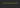# Convert string to integer in javascriptIn this tutorial, we are going to learn how to convert a numeric string to integer/number in javascript.

### First way

``````const a = "11";
console.log( + a ) // 11``````

if we just add the plus before the numeric string. it will convert as a number.

### Second way

Number object helps us to convert the numeric strings into a number.

``````const a = "11";
const b = 4;
const aplusb = Number(a)+b
console.log(aplusb) // 15``````

### Third way

`Number.parseInt()` method takes two arguments first one is string and second one is base

``````const a = "11";
const b = 4;
const aplusb = Number.parseInt(a,10)+b
console.log(aplusb) // 15``````

In the above code, we used the base 10 because of decimal numbers.

Happy coding…